Chapter 0.C. Models and Mathematics (Draft)

Introduction: For much of your studies in mathematics you have been told that we study mathematics because it has so many applications. Mathematics is important in a wide variety of disciplines and studies, from physics to ecology, from economics to sociology, from the stock market to the race track. Often the relationship of mathematics to its applications is described by the word "model".
• What is a model? When we think of models (outside of mathematics), several images may come to mind. You may think of model cars, dolls and doll houses, electric trains, ships in glass jars, or airplanes with remote control: the playthings of the young (in age or spirit). You may think of scaled preproduction structures used by designers, engineers, and architects for planning building, artistic, or engineering projects. You may imagine some fantastic computer-generated or holographic images that seem authentic as they appear in some "virtual reality." You may envision people in the fashion industry paid to wear clothing.  From these uses of the word model we can extract some common characteristics of a model. What follows is one attempt to identify some key elements of a model; you can try to write a description yourself or look in a dictionary for other ways to characterize a model. A model is a representation of an object or a context. A model presents some features of the original object or context so that some properties resulting from the specifically modelled features of the original object or context are also present in the model.
The extent to which the representation matches the original object or context in common properties provides some qualitative measure of the fit of the model to what it represents. Model cars and doll houses have many features that are the same except for scale as the real cars and houses they represent. Yet the engine in the model car is usually not a gasoline-driven motor with an electronic ignition system, and the doll house does not have plumbing with hot and cold running water. Models allow us to study how features of a complex object or system interact or react to change. In design and engineering, models help us understand, predict, and correct practical and aesthetic problems before setting out on more expensive tasks. Whether it be the best position for locating a hydroelectric dam or the relative size and quality of a wing on an airplane, a model helps creators make and test plans and decisions.
• What kind of model uses mathematics? For a model to use mathematics, the object or context that is being modelled must have some qualities that can be treated abstractly enough to fit in a mathematical structure. Commonly this means being able to measure something about the object with numbers. [Sometimes a model deals only with some ordered relation or connections that do not involve any quantitative measurement.] The abstraction needs to fit into some mathematical structure (either already studied or created just for the problem at hand). Then mathematical tools can analyze the structure, leading to new information relevant to the original object or context.

Examples of Models That Use Mathematics

Example 0.C.1.  Parked Cars. The mathematics in a model doesn't need to be very sophisticated. It can be as simple as a model for keeping track of how many cars are parked in a parking lot. Certainly what we need to do is count the cars that are there when we start out records and then keep a tally of the cars that enter and leave the lot.The simple statement that describes the relation for this model is that the number of cars in the parking lot at time t+k is the number of cars there at time t plus the number of cars that have parked between time t and time t+k less the number of cars that have left the lot during that same time period.

We can introduce some algebraic notation here. Let P(t) be the number of cars parked in the lot at time t, N(t,k) be the number of newly arrived cars that have parked between time t and time t+k, and L(t,k) be the number of cars that have left the parking lot between time t and time t+k. The rather simple abstraction relating these numbers would use the mathematical operations and structure of the number system. The model is then represented in the simple equation: P(t+k)=P(t) + N(t,k) - L(t,k). If we let the net change in the number of parked cars by DP = P(t+k) - P(t), the previous equation become DP=N(t,k) - L(t,k).

This simple (difference) equation model could be used just as easily to keep track of a population of birds in a forest, the amount of a water in a resevoir, or the amount of money in a checking account.

The fact that addition and subtraction in the number system works so easily and sensibly in representing the parked cars in the previous example may seem obvious. This next example demonstrates the choices made to process information in a model with mathematical structure.

Example 0.C.2. Batting Average. Suppose we are keeping track of a softball player's batting average during a ten-game season. In the first seven games the player was at bat 25 times and hit successfully 10 times for an average of 10/25= .400 (= 2/5). The batter had a small hitting streak during the last three games of the season with 6 hits for 10 times at bat, giving an average for those three games of 6/10=.600 (= 3/5).

How do we determine the player's batting average for the entire 10 game season? If the only thing we consider is that the information needs to be combined in some way, we have some choices to make.

We could add the two averages giving an average of .400 + .600 = 2/5 + 3/5=1.000. Of course that doesn't make sense, but how many times have you seen people just assume that the way to combine numbers is to add them?

Maybe we should average the two averages. If we follow this suggestion, we add the two averages and then divide the sum by 2, giving an average of .500=1/2. At least this sounds more reasonable as the kind of number that we would recognize as describing the batter's success rate. But this method also has problems. Haven't we given too much credit in the overall average to the last 10 at bats?

What makes more sense in finding the player's overall batting average is to determine the total number of the batter's hits and divide by the total number of times at bat. Following this procedure we find the batter had a total of 16 hits for 36 times at bat, or a batting average of .444 = 4/9. This is, in fact, the number that would be used to describe the player's batting average for the season.

What does this example show us about modelling with numbers? You need to look at the numerical information's meaning in the model to determine what arithmetic procedure will fit the desired operation. In the example, to add the batting information for a batting average we did not add the batting averages, but added separately the number of hits and at-bats to find the final batting average.

N.B. As simple as it may be, it is important enough to note again that the use of mathematical operations in a model needs some careful thought to see that it is appropriate for the context. These choices may require some subtle analysis of the object or context and the mathematics to have the model be accurate and consistent.

• What is the role of a mathematical model in an application? The purpose of a model in an application is to provide information about the object or context that is being modelled. A mathematical model allows the use of mathematical reasoning and operations leading to results in the mathematics which can be interpreted as statements about the object or context being modelled. Modelling questions that start in the real world can be translated into mathematical questions. The answers to these mathematical questions can be interpreted and applied to the original context. It is a back and forth process, like living in two worlds.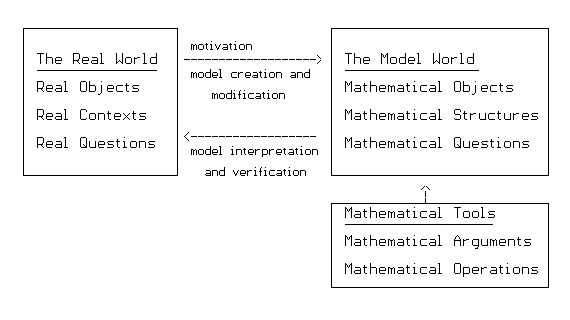So here's one way to think about mathematical models. In the real world we have objects, contexts, and questions. Motivated by the real world, we create a model world with mathematics in which many aspects of the real world are true. We examine the world of the mathematical model with the special tools of mathematics to answer the mathematical model questions that correspond to the real world questions. If we can answer these mathematical questions, we take those answers and interpret them as being sensible in the real world. (This is possible because of the correspondence we establish through language between the real world and the mathematical model.)

We use the model answers, translated into the real world, to understand the real world, make real decisions, and plan real projects. We also can revise our model to accommodate changes in the real world and inconsistencies between our initial model results and what we find upon further investigations in the real world.

More Models, Contexts, Settings and Functions.

Besides the trip context discussed in our discussion of functions in Chapter 0.B.2, there are many other common settings in which the function concept can be used to understand the relation between variable quantities in a mathematical model. Here are a few familiar and not so familiar situations that will appear throughout our discussions in this book.

Experiments, Randomness and Probability (darts). In an overwhelming number of sciences the most common context involves the repetition of an experiment. During the experiment observations are made to find relations between variables that are controlled and measured during the experiment. The relation between the variables may be considered deterministic, controlled almost completely by the interrelations of the variables, or probabilistic [sometimes called stochastic], seemingly unpredictable and occurring randomly based on the information known prior to the experiment.

You may or may not have had previous experience with the concepts of probability, so for the time being let's assume you have only an informal and somewhat naive view. In particular when we say that an event E has a probability of p (say, for example, p = 1/4) of happening, where p is some real number between 0 and 1 you may interpret this as meaning roughly that if an experiment is repeated a large number of times, say N times (for example, N=1000 times), then you might expect the event E will occur approximately pN times ( for the example, 1/4 1000 = 250 times). It is convenient to write the probability of event E is p with Pr(E)=p.

The nature of a random (stochastic) variable may be a little abstract, so let's use a simple model to illustrate some probability concepts we'll use later in the course.

Example 0.C.3: Darts. Imagine a circular board of radius 60 centimeters which is a powerfulmagnet. See Figure ***. This magnet is very strong so that when I turn my back to it and release a magnetic dart, the dart will be drawn to the board and will land at random somewhere on the board.

Let's assume the following uniformity condition for simplicity: If we have any two regions on the board of equal area then the dart would land in those regions about the same number of times with a large number of throws. In other words, the event of the dart falling in regions of equal area is equally probable or equi-likely.

I'll throw a magnetic dart and mark where it lands on the board. This is our basic experiment. Here are some typical questions for this dart experiment: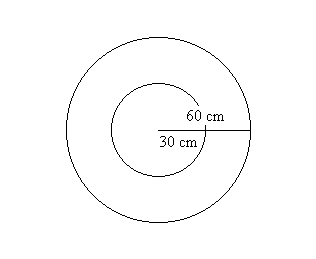(i) If the board is divided into two regions by a concentric circle of radius 30 and I repeat the experiment a large number of times, what proportion of the markings is likely to fall in the inner region? See Figure ***.

(ii) After repeating the experiment a large number of times, what proportion of the markings  is likely  to fall within A cms of the center where 0<A<60? See Figure ***.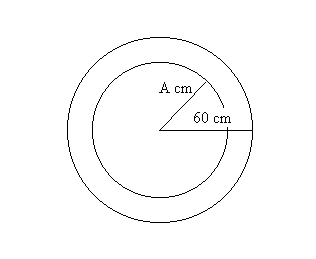(iii) If the board is divided into four regions by concentric circles of radius 15, 30, and 45 cm and I repeat the experiment a large number of times, what proportion of the markings from the experiments is likely to fall in
each of the regions?

(iv) If the board is divided into n regions by concentric circles of radius (k/n) 60 cms with k = 1,2 ... n-1, and I repeat the experiment a large number of times, what proportion of the markings from the experiments is likely to fall in each of the regions?

There are many observations that might be recorded after a throw. As in any experiment, we will measure something that helps us answer a question. For each of the questions we posed one important piece of information we can observe is the dart's distance from the center of the circle. This measurement varies unpredictably with each throw, so it is called a random variable of the experiment. We'll use the letter R to denote the value of this random variable measuring the distance of the dart from the center of the circle. Each of the questions translates into a corresponding question about the random variable R. After throwing the dart, the random variable is measured and we can answer any of questions of where the dart landed by examining the value of R.

For example, from question (i) we would like to know: what proportion of the experiments is likely to give a value of R less than 30, i.e., R<30. The general question for (ii) would ask: what proportion of the experiments is likely where the value of R is less than A, i.e. R<A.

The probability that the dart would fall within the circle of radius 30 is determined by finding the probability that R<30. After looking at Figure *** and some thought you should believe that this probability can be measured by finding the ratio of the area inside the circle of radius 30 to the area of the circle of radius 60. Thus the probability that R < 30 is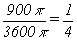.

Looking at the related question of the likelihood of the dart landing in the outer ring, we can analyze the areas of the regions in question to see the probability that 30<R is 3/4 .

Following the same type of reasoning, you should try to explain why the probability that R<A for any A between 0 and 60 will be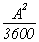while the probability that A < R for R between 0 and 60 will be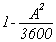. These results allow us to answer the nextQuestion: For what choice of A will the probability of the dart following inside the circle of radius A be the same as the probability that dart will land outside the circle? [The answer to this question can be described as the median value of R.]

Answer: Let's assume we have found such a value for A. Then our analysis shows that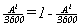, ... so after solving the algebra in this equation we can see that A must be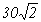. You can check that this value for A does answer the question correctly. See Figure ***.Extension: A slightly more interesting question is where to place two concentric circles so that the probabilities of the dart landing in either the inner circle, the outer ring, or the central ring are all equal (namely 1/3). See Figure ***. From the previous discussion on the probability of the dart falling inside a circle of radius A we have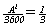and likewise from the probability of the dart falling outside the circle of radius B we have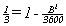. With these equations we can conclude that A = 20sqrt(3) and B = 20sqrt(6).

The only additional general analysis we need to settle the third part of this question is the probability of the dart landing inside the ring bounded by concentric circles of radius B and A (assuming that B> A). As before we can use the ratio of areas to measure the probability and you can check that this quantity is precisely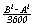. This formula works out to be 1/3 with the previously found values for A and B, so those values do make all three regions have equal area.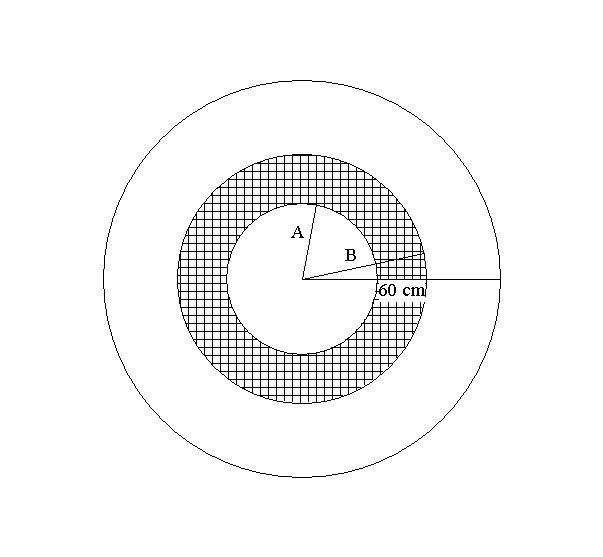Notation: It is helpful and traditional to denote the probability that a random variable R has a value <= A by F(A) where A in this case is in the closed interval [0,60].
F is a function of A defined on the interval [0,60] and is called a cu
mulative probability distribution function for the random variable R, in short, the distribution function for R. In fact, though it may seem strange, we can extend the domain of the function F to all real numbers. This function can be analyzed by cases so that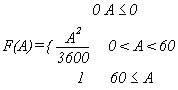.
See the figure for a graph of this function. You should sketch a transformation figure for this function yourself.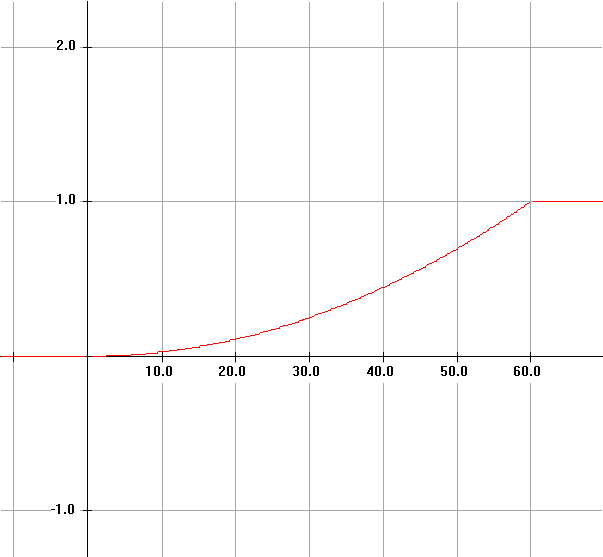Probability Density: We continue our examination of the circular dart board in the next example with a different focus. We'll still use R as our random variable reporting the distance of the dart from the center of the circle. The area analysis we did previously shows that the probability of the dart being in a very thin ring would be close to 0 and thus that the probability of the dart landing precisely on any specified value, say R = 30, would be 0. Yet when we consider the probability that R lands between 10 and 20 , we find it is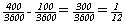. Comparing this to the probability of landing between 40 and 50, which is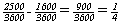, suggests that the latter event is three times more likely to occur. See Figure ***.

The issue is to explain why values for R are distributed in a non uniform way and to give some measure for the likelihood of R being close to or equal to a particular value. We'll analyze how the interval in which the R occurs controls the likelihood of its occurrence, depending on the value of the R and the length of the interval in which the R lands. To do this we need to compare probabilities with interval length. The concept of average probability density was developed to do just that. The average probability density (APD) of a random variable R for an interval [A,B] is the ratio of the probability that A < R < B to the length of the interval [A,B]. Using the probability distribution function F we have that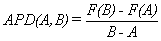.

Here's the continuation of our example. We find the average probability density for R for the intervals [10,20] and [40,50]. Using the probabilities from the previous discussion the average probability density for [10,20] is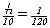while the APD for [40,50] is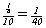.

Economics Models. It is practically impossible today to ignore the economic world that surrounds our lives. The science of economics studies more than business. Its studies extend to fundamental questions of the development and allocation of the physical and personal resources we use and don't use. Economic issues of large scope and effect, like national income and taxation, government spending, national employment, and bank interest rates are usually described by the term macroeconomics. Those of smaller scope, but not necessarily of lesser effect or importance, like business planning, industry growth, market supply and demand, profit, and prices, are characterized as microeconomics.

All of economics is interested today in accurate understanding of the current state of economic affairs and the possible future changes to that state. To be a little more concrete, economics is interested in the large scale effects of a change in taxation, in the interest rate charged to large borrowers, and in consumer spending, as well as the relatively smaller effects of a rise in the price of a pound of butter, a military base closure, or a court ruling on the environment. In Chapter 1 we will look at what economist call marginal analysis, one of the general conceptual tools used to analyze both macro- and micro- economic decisions. The margins of a page refer to the extra space near the edge of the page reserved for something other than the main text. In economics, marginal has a more technical but related meaning. At this point we will look only at some simple economic models in which this type of analysis develops.

In examining contexts for which economic analysis is used for evaluation and decision making the variables that are measured usually concern the values of costs and benefits. In a business enterprise there are many costs of production, such as costs for raw materials, tools, labor, costs of sales and distribution, such as transportation, advertisement, packaging, and more labor, and costs after sales, such as service and product liability. Benefits in business are usually financial, found through an accounting procedure that evaluates income and assets. In the public sector of an economic system there are also costs and benefits for providing services such as roads, sewers, water, and other infrastructure for the functioning of society as well as police, fire, schools, and other social services.

Example 0.C.4. Umbrellas: As a simple example of a model used in a small business context, let's consider The Umbrella Company (TUC), a small company that manufactures umbrellas with artistic prints on the fabric. For about 6 months TUC had been producing 1000 umbrellas in a month at a cost of \$3000. It sold the umbrellas wholesale to BIG Stores, a large department store chain, and sales went well so BIG purchased every umbrella TUC had produced at \$7 per umbrella and also paid the shipping costs. These sales produced \$7000 in revenues per month for TUC and after subtracting the costs, an income from sales of \$4000 per month. The average cost was \$3 per umbrella and the average profit was \$4 per umbrella.

In July TUC decided to increase production slightly and produced 1050 umbrellas. The total costs increased to \$3100 and BIG bought the extra 50 umbrellas at the same price of \$7. The TUC owners were happy about the change since it appeared that the additional 50 umbrellas had cost on average only \$2 per umbrella. Thus while lowering the overall average cost to \$3100/1050 or approximately \$2.96 per umbrella, this small change in production brought a \$400 change in profit, \$100 more than they might have expected. Based on this experience TUC increased their production plan to produce 1050 umbrellas every month.

Adding a little notation and technical language to the description of the TUC story will give a little more mathematical appearance to the model. Let Dx denote the additional 50 umbrellas produced and DC denote the increase of \$100 in total cost resulting from the increased production. So each additional umbrella cost \$2 instead of \$3. The ratio of change in cost to change in level of production is called the average marginal cost (with respect to production). When the units of production are indivisible, like an umbrella, the marginal cost is the added cost for producing one more unit- one more umbrella. In general the average marginal cost, MC, is defined by the ratio of the change in cost, DC, divided by the number of additional units produced, Dx, so MC = DC/Dx. In the umbrella example we had MC = \$100/50 umbrellas = \$2/umbrella when producing 1000 umbrellas. If we assume the marginal cost stays the same for some range of values for Dx, and that the price and sales of umbrellas remain the same as well, we can use this model to predict how profits from umbrella sales would change from other possible changes in the level of production. It would allow prediction of the effect on average costs and profits as well.

Population Models. When we speak of a population, we usually think we are discussing some biological entity, humans, mammals, animals, vegetation, even as small as a virus or an amoeba. These populations change and we are concerned with how to measure them, how to predict their size in the future, and how to control their growth or bring an end to their existence. Some of these populations are measured only with counting numbers, as we describe the number of humans, dogs and cats, or owls with variables. Sometimes we describe the populations with real numbers, as with the weight of the harvest of tomatoes or the catch of fish. The modelling questions are those of continued growth with or without bound, diminishing size and possible extinction. We are concerned and wish to understand what might control any patterns of growth that appear cyclical and what may limit or contain the growth of populations beyond or below a desirable size. The study of sub-populations are also quite important. We may want to keep track of those members of the population that are infected with a certain virus for public health studies, or those that have incomes over \$100,000 for economic studies, or those that live in a certain type of ecosystem for environmental studies.

Learning Models. We all have some experience with learning. How do we measure learning? We establish some activities and measurable performance criteria which we believe are connected to learning. Some of these use counting numbers, such as how many questions a person can answer correctly on a test. Others are measured with real numbers, like the time it takes to complete a task, or the number that results from averaging several evaluations of the quality and quantity of learning of an individual or a group. Our questions for a learning model depend on the context. Will an individual's learning increase steadily over time? Is there a bound to an individual's ability to learn and if so how can we predict what it will be? How do process comprehension and fatigue affect the rate of learning? Are the features of individual learning different from group learning processes?

Water and other fluid models. Water (or any fluid) can be thought of as flowing in and out of a container. This may be a glass or goblet, a sink or bathtub, a hot tub or a bathing pool, a water tank or a reservoir, a pond or a marsh. We measure the volume of the water, the surface area of the water, the height or depth of the water or the amount of impurities present in a one liter sample. All of these measurements can be thought of as functions of time, and depending on the situation many of these variables can be considered as functions of the others. Thus we might easily see that the height of the water above a fixed marker as a function of the volume of the water, or vice versa, that the volume of the water is a function of the height of the water. Our interests depend on our concerns. What are the levels of impurities in the water for environmental protection, or at what rate is the water flowing in or out for overflow and flooding predictions.

Temperature, Pressure, Humidity, ..., The Weather. The weather has been a subject of study ever since it has affected the way humans interact with their environment. Newspapers commonly devote about a page every day to numerical information about the weather. Though you don't need a weatherman to know which way the wind blows, information about changing temperatures, pressure, humidity, and other measurements of the atmospheric conditions all contribute to our ability to understand and predict the weather. And with prediction we can plan to control and limit the more severe results of the weather, be it in preparing for droughts, floods, extreme heat, frigid cold, or high winds.

Health and Medicine: Speaking of temperatures brings to mind the famous 98.6 degrees body temperature. The study of the human body for both medicine and surgery has developed models for understanding and preventing diseases, correcting and repairing malfunctioning organs, and enhancing or preventing reproduction. The periodic behavior of many human systems from the pumping of the heart to the variations in body temperatures all suggest that mathematical models for these systems might involve trigonometric functions like the sine and cosine. And certainly we look to many measurements of rates and pressures under various conditions to indicate other aspects of the health of our bodies.

Sports. The world of sports may seem shallow in comparison with some of the issues we've mentioned already in trying to introduce mathematical models related to your own experience. You don't have to be a famous athlete to participate in sports whether for personal enjoyment or the excitement of competition. Whether its running, swimming, or jumping, skate boarding, surfboarding or snowboarding, riding a bicycle, paddling a canoe, or riding a sled, playing soccer, or water polo, of hockey, throwing a softball, a basketball, or football, whatever the activity, there are a wealth of studies using mathematics that suggest ways to improve your performance.

Exercises 0.C.

1. For the random variable R of Example 0.C.3 find probabilities for the following events:

2. a) R <= 18 b) R <= 42 c) 18 <= R d) 18 <= R <= 42 e) 18<=R <= 20
3. For the random variable R of Example 0.C.3 find the radii for three concentric circles so that the dart is equally likely to fall in the center circle or the three rings the circles determine in the unit circle. Discuss how you would solve the similar problem for four circles. Make a conjecture for the problem with n circles.

5. For the random variable R of Example 0.C.3 find the average probability density for the following intervals: a) [18,42] b) [18,24] c) [18,19] d) [12,18] e) [17,18]

6.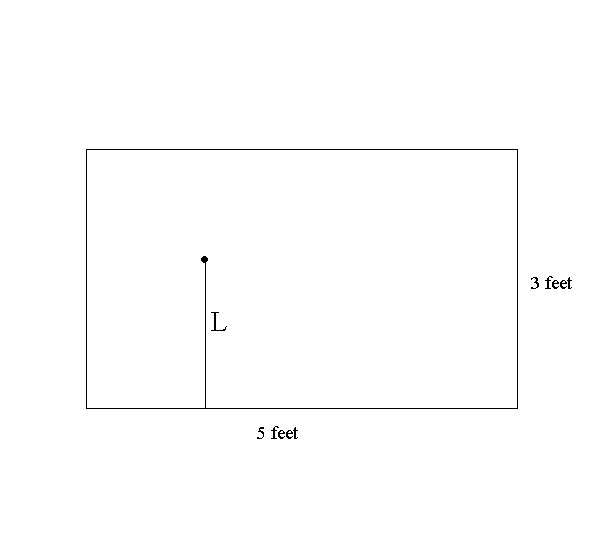7. Suppose the dart board for our experiment is a rectangle 3' by 5' in dimension and when the dart lands we report the distance of the dart to one predetermined 5' side with the random variable L. [See Figure I.18]

8. a) Let F(A) denote the probability that L < A where 0 < A < 3. Find F(1) , F(1.5) , F(2), and F(A). [Hint: For what region will L < 1? ] Use a transformation figure and a graph to visualize F.

b) Find the probability that 1 <= L <= 2. Find the average probability density for L on the following intervals:

i) [1,2],            ii) [1,1.1]               iii) [.9, 1].

9. Suppose the dart board is a right triangle with legs 3' and 4' and when the dart lands we report the distance of the dart to the 4' side with the random variable H. [See Figure I.19]10.

a) Let F(A) denote the probability that H < A where 0 < A < 3. Find F(1) , F(1.5) , F(2), and F(A). [Hint: For what region in the triangle will H<A?] Use a transformation figure and a graph to visualize F.

b) Find the probability that 1 <  H < 2. Find the average probability density for H on the following intervals: i) [1,2], ii) [1,1.1] iii) [.9, 1].

In problems 6 through 10, suppose X is a random variable which takes values in the interval [0,2] and that F(A) gives the probability that X <= A where 0<A<2 . Use a transformation figure and a graph to visualize these functions.

a. Find the average probability density of X in the intervals [1,2], [1,1.1], [0,1], and [.9,1].

b. Find a number A where 0 < A < 2 where the probability that X < A is the same as the probability that A < X .

11. F(A) = .5 A.
12. F(A) = 1/4 A 2 .
13. F(A) = A 2 - 3/2 A.
14. F(A) = 1/8 A 3 .
15. F(A) = 1/16 A 4.
16. Suppose F(A) = sin(A) describes the probability that a random variable Y is less than or equal to A where 0 < A < p/2. Using your calculator estimate the average probability density for Y for the intervals [p/6, p/4] , [p/4, p/3], and [p/4, p/4 + .1].
17. Suppose that an object is inside a sphere of radius 10 centimeters and it is equally likely that the point is at any position in the sphere. Let R denote the random variable that measures the distance from the object to the center of the sphere. Describe the (cumulative) probability distribution for R, that is, the function F where F(A) is the probability that R < A with 0< A<10? Use a transformation figure and a graph to visualize F.
18. Darts and Averages. (Project) Consider a circular magnetic dart board with radius 60 centimeters as described in Example 0.C.3. Draw 5 concentric circles on the board with radii 10,20,30,40, and 50 creating 6 regions (one disc and five bands). See Figure ***. Here is our game: We will throw the dart at the board and score 5 points for landing in the inner disc, 15 points for landing in the smallest band ( between the circles of radius 10 and 20), 25 points for landing in the next smallest band ( between the circles of radius 20 and 30), 35 points for landing in the band between the circles of radius 30 and 40, 45 points for landing in the band between the circles of radius 40 and 50, and 55 points for landing in the outermost band (between the circle of radius 50 and and the edge of the dart board). [In our game a low score would be considered evidence of greater skill.]

19.

We allow a player to throw a dart 36 times and find the total score for the player as well as the average score (the total divided by 36). [The average will be a number between 5 and 55.] Give a total score and an average that you might expect for a player with no special skill. [These numbers might be called the expected score and the expected average for the game.] Discuss your reasoning and show your work leading to your proposed solution.

Suggestion: You might investigate the similar problem with 2 regions and 4 throws and then 4 regions and 16 throws as a way to begin thinking about the problem. How many darts do you think would fall in each region?

Extension: For each of the 36 throws, suppose we measure the distance the dart lands from the center. What do you think the average distance would be for a player with no special skills. Explain your reasoning, any connections with the expected average score in the game, and whether you have any belief about the accuracy of your response. (Is it an underestimate or an overestimate?)

20. The Umbrella Company has started a new line of small collapsible umbrellas that seem to be selling quite well at \$4 per umbrella. For the first three months TUC produced 500 umbrellas per month at a total cost of \$1500 per month. In the fourth month TUC had to lower production to 450 umbrellas with a resulting reduction in costs for that month to \$1400. The manager thinks this information can be used to justify increasing production to 550 or even 600 umbrellas per month since the sales people say the demand for these umbrellas will support that level of production. Use a transformation figure and a graph to visualize this information. Do you agree? Explain your response using a mathematical model that discusses costs, revenues and profits. What assumptions in your model are supported by the information and what assumptions are subject to some doubt?

21.
22. The Stock Market. The ability to predict prices on the stock market is one of the keys to making a fortune through the exchange of stocks on the public stock markets. Choose two or three stocks related to local companies that are reported in your daily newspaper and keep track of these prices and any other news reported about these companies for two weeks. Use a transformation figure and a graph to visualize your information. Can you find any patterns in the data that suggest a mathematical formula for predicting the opening price of a stock for one day based on the prices for the previous two days? What difficulties are there in creating such a formula? What about a formula based on the previous three days?

23.
24. The Weather. Using local weather information for two weeks, can you find any patterns in the data that suggest a mathematical formula for predicting the temperature at noon for one day based on the temperature at noon for the previous two days? [Use a transformation figure and a graph to visualize your information. ] What difficulties are there in creating such a formula? What about a formula based on the previous three days?

25.
26. Temperature: Human body temperature is often considered a key indicator of body functions. Many people keep track of their daily temperature by measuring it each morning upon awakening. This kind of record keeping usually requires a fair amount of discipline and routine. Can you suggest some ways in which this information would be useful?

27.
28. Sight and Hearing. Consider the senses that we commonly use to experience our surroundings.
1. In a model for visual experience what variables would you study?
2. In a model for aural experience what variables would you study?